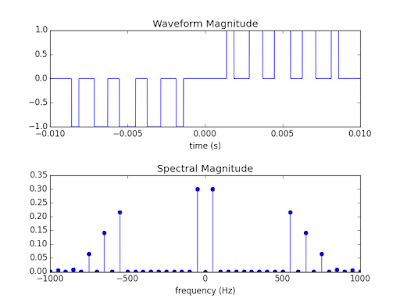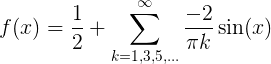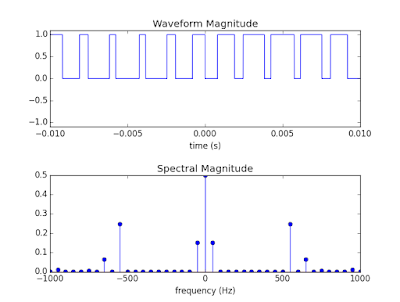## Thursday, October 8, 2015

### I hope you find this blog enjoyable and helpful. Support it with a small donation.

A couple years ago I wrote some code to generate Harmonic Elimination PWM (HEPWM) waveforms in octave, which is kind of like Matlab.  Recently someone asked if I could rewrite it in Python so it'd be easier to use and understand (fair call, it was pretty archaic).  I jumped at the chance as I'd been meaning to do it but just didn't have a strong reason to.  While I was at it I added a feature that may make it more useful, but more on that later.  To understand everything I recommend reading my previous HEPWM posts.

I should take a step back and first explain what HEPWM waveforms are.  If you're reading this I'm going to assume that you know what a PWM wave is and that you know the square nature of periodic PWM waves generate unwanted harmonics.  HEPWM is an extension of that idea, but the timing of the transitions in the wave are calculated to cancel or reduce specified harmonics.  The even harmonics are eliminated by default by making the wave half wave symmetric.  You don't have to make the wave quarter wave symmetric, that's a bit of a maths trick, but by doing that you halve the number of equations and unknowns that you need to solve for.  (If I remember correctly, solving the entire set of equations allows you to control the phase of the harmonics as well.)  That sounds really complicated, but the image below may help.

The upper waveform is typical of what you'd see on the output of an unfiltered inverter.  In this case the period of the wave is 20 ms, which means the fundamental frequency is 50 Hz.  The HEPWM calculations however have allowed the creation of a wave with the 3rd, 5th, 7th, and 9th harmonics set to zero and the fundamental frequency set to 0.6 (It looks like 0.3, but you have to remember that the positive and negative frequencies add in this case)  As we're controlling 5 harmonics (1, 3, 5, 7, 9) there needs to be 5 transitions in each quarter of the waveform.3 Level HE-PWM
The HEPWM waveform is constructed by starting with the base waveform below.  Multiple versions of this waveform are alternatively added and subtracted with the alpha values increasing each time (Alpha values are always between 0 and T/4)Quarter Wave Symmetric Waveform
Start with the definition for the Fourier series below.  It can then be applied to the above waveform.Fourier Series
If you do the math X = 0, B[k]=0 and A[k]=0 for even positive values of k.  For positive odd values of k, A[k] is shown below.Fourier Series Coefficient for QWS waveform
This is the magnitude of a particular harmonic, k, for a particular value of alpha.  The total value for each harmonic can be found by adding all the components from each value of alpha (remember alternating alpha values cause the sign to change).  It helps to note the relation between the period and angular frequency.  Combining this with the above equation a system of equations can be generated and solved.  I go into more detail in previous posts, but this is needed to give context to the next addition.Angular Frequency
The 3 level waveform in the first image is easy to generate with a H bridge topology, but what if you want to accomplish a similar effect with a microcontroller with only 2 output levels?  It seems that you can just shift the first half of the waveform up by adding the waveform below, but by doing that you are adding the Fourier series of the below wave to the Fourier series of the waveform calculated above.  This will invalidate the magnitudes of the harmonics you are trying to target.  I reused some images from a previous post that used a period of T but it makes more sense to use a generalised period of 2 pi.Translated Square Wave
All is not lost though, calculating the Fourier series of translated square wave above gives the result below.  There's nothing we can do about the DC offset, but the summation term can be easily added to the  calculated harmonics of the original waveform because it only has a sine term.  All we need to do is tweak the code the generates the set of simultaneous equations to be solved and we can now move to a 2 level system.Fourier series of Translated Square Wave
For clarity, a two level system with the 3rd, 5th, 7th, and 9th harmonics nulled and the main harmonic set to 0.3 is shown.  The main difference between the spectrum of this waveform and the one above is the DC offset.  That's what coupling capacitors are for.2 Level HEPWM
Below is the python code that does this little trick.  When the twoLevels variable is set to 0, the code runs as usual and generates the transition points for a 3 level waveform.  When it's set to 1, the adjustment term that describes the Fourier series of the square wave is included in the calculations.  (What the hell was I thinking with that comment "the location of the switching location")Calculating the Harmonic Magnitude in Python

Caveats, Warnings and Errata

This code isn't meant to hold your hand.  It's a demonstration and will probably have errors.  If you find any let me know.  It also assumes that your waveforms have perfect 0 time transitions with no overshoot or similar real world effects.  I recommend using it as a starting point.  Calculate your transition points and do a localised search with those values while running simulations closer to the real world.

I did my best to document things, but it's hard to comment symbolic math equations.  I may look into that.Get the code!